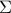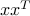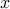# On the Quality of a Semidefinite Programming Bound for Sparse Principal Component Analysis

• Author: L. El Ghaoui.

• Status: Preprint on arXiv.

• Abstract: We examine the problem of approximating a positive, semidefinite matrixby a dyad, with a penalty on the cardinality of the vector. This problem arises in sparse principal component analysis, where a decomposition ofinvolving sparse factors is sought. We express this hard, combinatorial problem as a maximum eigenvalue problem, in which we seek to maximize, over a box, the largest eigenvalue of a symmetric matrix that is linear in the variables. This representation allows to use the techniques of robust optimization, to derive a bound based on semidefinite programming. The quality of the bound is investigated using a technique inspired by Nemirovski and Ben-Tal (2002).

• Bibtex reference:

```@unpublished{Elg:06,
Author = {L. {El Ghaoui}},
Month = {February},
Note = {arXiv:math/060144},
Title = {On the Quality of a Semidefinite Programming
Bound for Sparse Principal Component Analysis},
Year = {2006}}
```